StatLect

# Values of the normal distribution

In this lecture we discuss how to compute the values of the normal distribution function, using normal distribution tables or computer programs (in particular Matlab or Excel).## Values of a standard normal distribution

Letbe a standard normal random variable (i.e., a normal random variable with zero mean and unit variance) and denote its distribution function by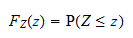As we have discussed in the lecture entitled Normal distribution, there is no simple analytical expression forand its values are usually looked up in a table or computed with a computer algorithm.

This lecture tackles the practical problem of computing (numerically) the values ofwhenis a specific number. We can confine our attention to standard normal random variables: as shown in the next section, if we know how to compute the values of a standard normal distribution, we also know how to compute the values of any other normal distribution.

### Frequently used values

Some values of the normal distribution function are used very frequently and people usually learn them by heart: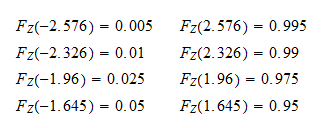### Symmetry

A fact that is often used in calculations is the following: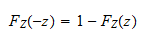This is due to the symmetry around 0 of the normal density.

## Values of a non-standard normal distribution

Letbe a normal random variable with meanand varianceand denote its distribution function by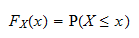Remember that any normal random variablewith meanand variancecan be written as:whereis a standard normal random variable.

Using this fact, we obtain the following relation between the distribution function of a standard normal random variableand the distribution function of any other normal random variable:Therefore, if we know how to compute the values of a standard normal distribution, we also know how to compute the values of a normal distribution with meanand variance.

Example If we need to compute the valueof a normal random variablewith meanand variance, we can compute it using the distribution function of a standard normal random variable: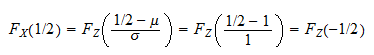## Normal distribution tables

In the past, when computers were not widely available, people used to look up the values ofin normal distribution tables, wherewas tabulated for several values of. A normal distribution table looks something like this:

z F(z) z F(z) z F(z)
0.00 0.5000 1.00 0.8413 2.00 0.9772
0.05 0.5199 1.05 0.8531 2.05 0.9798
0.10 0.5398 1.10 0.8643 2.10 0.9821
0.15 0.5596 1.15 0.8749 2.15 0.9842
0.20 0.5793 1.20 0.8849 2.20 0.9861
0.25 0.5987 1.25 0.8944 2.25 0.9878
0.30 0.6179 1.30 0.9032 2.30 0.9893
0.35 0.6368 1.35 0.9115 2.35 0.9906
0.40 0.6554 1.40 0.9192 2.40 0.9918
0.45 0.6736 1.45 0.9265 2.45 0.9929
0.50 0.6915 1.50 0.9332 2.50 0.9938
0.55 0.7088 1.55 0.9394 2.55 0.9946
0.60 0.7257 1.60 0.9452 2.60 0.9953
0.65 0.7422 1.65 0.9505 2.65 0.9960
0.70 0.7580 1.70 0.9554 2.70 0.9965
0.75 0.7734 1.75 0.9599 2.75 0.9970
0.80 0.7881 1.80 0.9641 2.80 0.9974
0.85 0.8023 1.85 0.9678 2.85 0.9978
0.90 0.8159 1.90 0.9713 2.90 0.9981
0.95 0.8289 1.95 0.9744 2.95 0.9984

For example, to computewe need to search for the row corresponding to the value. We find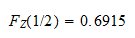If we are searching for a value ofthat is not tabulated, we can compute an approximation ofby interpolating the two values that are closest to. For example,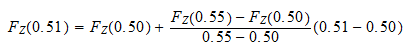Note also that the table does not contain the values of the distribution function corresponding to negative values of, but these values can be derived using the symmetry of the normal distribution (see above).

## Normal distribution values in Excel

To compute the values of the normal distribution function, we can use the built-in Excel function `NORM.S.DIST()`. For example, if we need to computeand the valueis stored in cell `A1`, we can type in another cell:

`=NORM.S.DIST(A1)`

## Normal distribution values in Matlab

To compute the values of the normal distribution function, we can use the Matlab function `normcdf()`. For example, if we need to compute, we can input the following command:

`normcdf(0.5)`

## Solved exercises

At the end of the lecture entitled Normal distribution, you can find some solved exercises that also require the computation of normal distribution values.